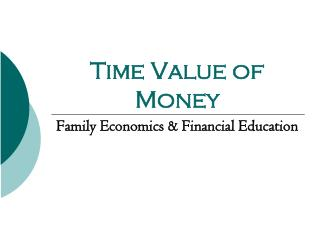DownloadDownload PresentationTime Value of Money

# Time Value of Money

Download Presentation## Time Value of Money

- - - - - - - - - - - - - - - - - - - - - - - - - - - E N D - - - - - - - - - - - - - - - - - - - - - - - - - - -
##### Presentation Transcript

1. Time Value of Money Family Economics & Financial Education

2. Time Value of Money • Time value of money – Because you can receive interest on any money you have, money received today is worth more than money received in the future • For example, if you have \$1000 today and receive 5% interest, it will be worth \$1050 in a year • If you receive \$1000 in a year, however, it is only worth \$1000

3. Compounding Interest • Compounding interest -- Earning interest on interest • “Make your money work for you” • Developed because compounding interest causes money to make money (notice that you get more in interest every year because you are receiving interest on your original investment and also on the interest you have earned)

4. Simple Interest • Simple interest-- Interest earned on the principal investment (not compounded) • Principal-- The original amount of money invested or saved • Amount invested x annual interest rate x number of years = interest earned (notice that you receive less in interest compared to the previous compounding example) • Ex. 1,000 x 0.10 x 2=\$200

5. Three Factors Affecting the Time Value Calculations • Time • Amount invested • Interest rate

6. Time • The earlier an individual invests, the more time their investment has to compound interest and increase in value

7. A Little Goes a Long Way • Sally Saver puts away \$3,000 per year in her IRA account earning 10% starting at age 18- she does this for 10 years then stops. • Sally accumulates \$1,239,564 by the age of 65. • Ed Uninformed waits until he is 28. He must contribute \$3,000 to his IRA account earning 10% for 38 years. • Ed accumulates \$1,102,331 by the age of 65

8. Amount Invested • Investing only a small amount a month is better than not investing at all • Ex. At 8% interest, invested at age 17, one dollar per day will become \$17,865.52 by age 65 • The larger the amount invested the greater return a person will earn • Always pay yourself first • Savings should be a fixed expense

9. Amount Invested continued • 70-20-10 Rule • 70% Spent • 20% Saved • 10% Invested • Flexible expenses can be decreased in order to increase the amount a person is able to invest

10. The Costs Add Up Investing at age 18 at 8% interest until age 65.

11. Interest Rate • The percentage rate paid on the money invested or saved • Higher interest=more money earned

12. Risk • A higher interest rate generally has a greater risk • Risk -- The uncertainty of the outcome of an investment • Example: Investing money in the stock market generally provides a high return, but it is riskier—you may lose more money than you gain

13. Fixed Interest Rate • Fixed interest rate-- The rate will not change for the lifetime of the investment • Having a savings or investment plan with a fixed interest rate guarantees a specific return but can provide a moderate risk • If the average interest rates rise, the amount a person earns from this type of investment will not increase

14. Inflation • Another consideration with interest rates is ensuring the interest rate is higher than the rate of inflation • Inflation-- The steady rise in the general level of prices • Example: If an individual has money invested at 4% interest and the inflation rate is 4%, the individual’s wealth will stay the same

15. Time Value of Money Calculations • Present value • PV=(FV)(1+i)-N • Future value • FV=(PV)(1+i)N • Financial calculators may be used to complete these calculations.

16. Calculation Components • Present value (PV)-- How much money a person has today • Future value (FV) – How much money a person expects to have in the future • Interest rate (i) – The percentage rate paid on the money invested or saved • Time (N) --Length of investment • Calculated by the number of compounding periods (daily, monthly, or annually)

17. Review • Compounding interest earns interest on interest • Increased time=more interest earned • Higher principle=more interest earned • Higher interest rate=more interest earned